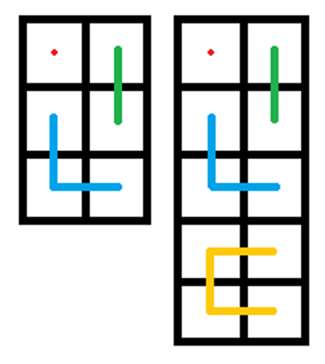# 4553. Snake

Setsuna is an expert of Gluttonous Snake, and for that reason she started a Gluttonous Snake club!

One day she was inspired by an obscure group logo (below) and needed a few twisted snakes for her club’s logo.She wants you to design an ideal association logo for her.And the logo should satisfy:

• There are exactly $n$ snakes in the logo and the $i$-th snake should cover exactly $i$ grids.
• All snakes are located on grids of $h$ rows and $w$ columns, where $h,w(hw=\frac{n(n+1)}{2})$ are two appropriate positive integers decided by yourself. Each grid should be covered by exactly one snake.
• Each snake is continuous on its own, and the $i$-th snake occupies a total of $i$ sequentially adjacent grids (two grids are defined to be adjacent if and only if they have a common side).
• No snake can pass through itself.
• Except for the $1$-st and the $2$-nd snakes, snakes covering even grids need to have an even number (excluding $0$) of turns , and snakes covering odd grids need to have an odd number of turns. And a turn means a $90^\circ$ turn. (see the example logos).

For example, the following diagrams are valid solutions for $n=3,4$.### 输入格式

Only one integer, $n$, indicating the number of snakes.

### 输出格式

If no legal logo exists, output a line “0 0”.

Otherwise, output two integers $h$ and $w$ on the first line, separated by a space, indicating the number of rows and columns of the grids. The coordinate of the grid at the upper left corner is $(1,1)$, while the coordinate of the grid at the lower right corner is $(h,w)$.

Then follow $n$ rows, the $i$-th row contains $2i$ integers separated by spaces, indicating $i$ coordinates of the grids occupied by the $i$-th snake, from its head to tail (see the sample).

The answer is not unique, and you can output any valid solution. Your answer must satisfy all of the following conditions to be considered correct.

• $h\times w=\frac{n\times (n+1)}{2}$

• The $h\times w$ coordinates $(x_i,y_i)$ in output should be pairwise different and $1\le x_i \le h,1\le y_i \le w$.

• For the $i$-th snake, its $i$ coordinates are $(x_1,y_1),(x_2,y_2),\cdots,(x_i,y_i)$, and for all $1\leq k < i$ needs to satisfy one of the following two conditions.

• $|x_k-x_{k+1}|=1 \text{ and }\ y_k=y_{k+1}$

• $x_k=x_{k+1}\text{ and }\ |y_k-y_{k+1}|=1$

• The number of turns of the $i$-th snake is defined formally as $\sum\limits_{k=2}^{i-1}[\neg( |x_{k-1}-x_{k+1}|=2\vee\ |y_{k-1}-y_{k+1}|=2 )]$, whose parity is subject to the $5$-th restriction of the above description. (You can just refer it as the number of $90^\circ$ turns in logo.)

Note that: Don’t print any extra space at the end of any line.

### 样例

Input
3

Output
3 2
1 1
2 2 1 2
3 2 3 1 2 1


### 提示

$1\leq n \leq 500$

18 人解决，31 人已尝试。

19 份提交通过，共有 146 份提交。

6.0 EMB 奖励。